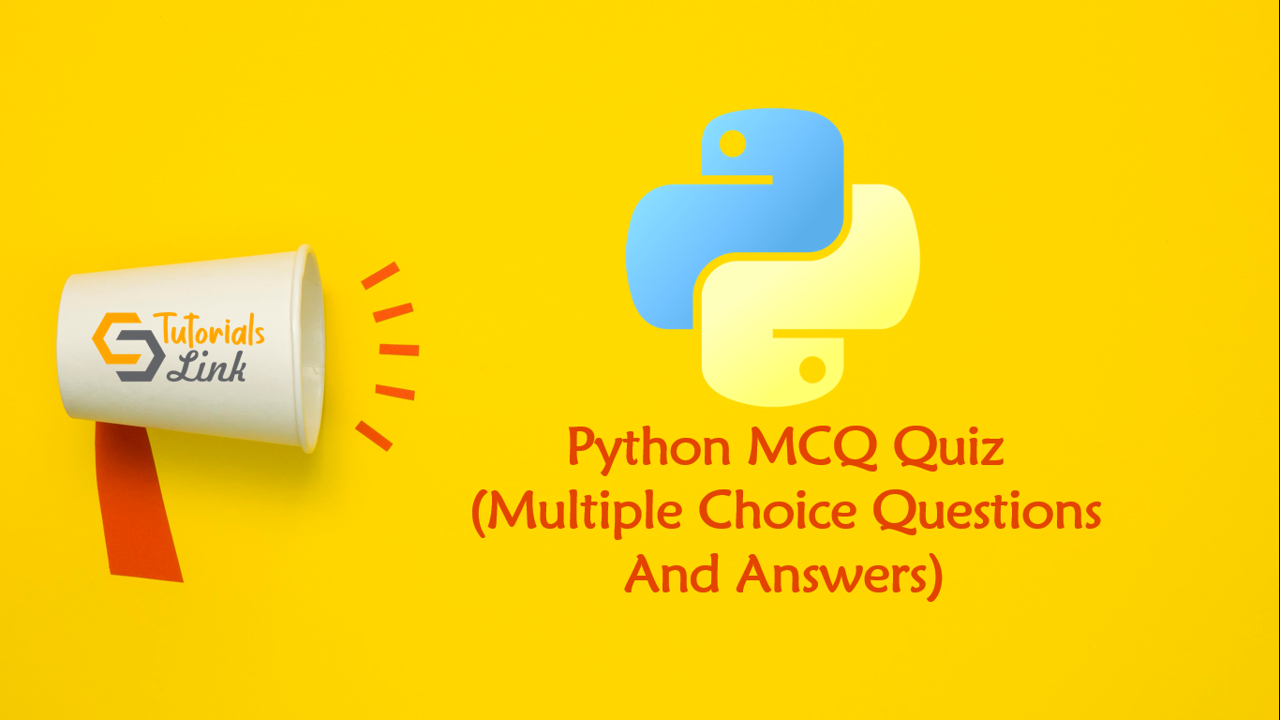Loading, please wait...# Python Multiple Choice Questions And Answers

Search here for MCQs

Are you preparing for the next job interviews? If yes, trust me this post will help you also we'll suggest you check out a big collection for Programming Full Forms that may help you in your interview:

## 46. Assume the output

values = [[3, 4, 5, 1], [33, 6, 1, 2]] v = values for lst in values: for element in lst: if v > element: v = element print(v)
• 3
• 1
• 2
• 33

## 47. Output is

x = [i**+1 for i in range(3)]; print(x);
• [0,1,2]
• [1,2,5]
• Error ';"
• None of the above

## 48. Predict the output

List = [True, 50, 10] List.insert(2, 5) print(List, "Sum is: ", sum(List))
• [True, 50, 10, 5] Sum is: 66
• [True, 50, 5, 10] Sum is: 65
• [True, 50, 5, 10] Sum is: 66
• TypeError: unsupported operand type(s) for +: ‘int’ and ‘str’

## 49. Output is

l = [1,2,6,5,7,8] l.insert(9)
• l=[9,1,2,6,5,7,8]
• Error
• l=[1,2,6,5,9.7,8] (insert randomly at any position)
• l=[1,2,6,5,7,8,9]

## 50. Assume the output

a=list((45,)*4) print((45)*4) print(a)
• 180[(45),(45),(45),(45)].
• (45,45,45,45).[45,45,45,45].
• 180[45,45,45,45].
• Syntax error